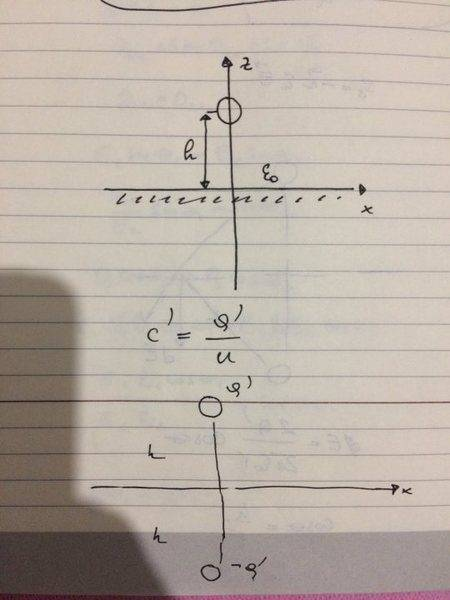# Determining the capacitance of the two conductors

## Homework Statement

For a given system, a conducting cylinder with radius ##r=a## with a linear charge density ##Q'## and a conducting surface at a distance ##z=h## from the cylinder, calculate the linear capacitance of the cylinder.Take that ##h>>a##
##C'=\frac{Q'}{U}#### Homework Equations

3. The Attempt at a Solution [/B]
This is my first thread on physicsforums so you tell me if i break any rules ok :D?
As shown in the image above, i took a mirror image of an object and charged it with a negative sign of the given charge. All i need to find is the potential from the cylinder to the image cylinder right?
The total electric field along the line i am integrating the potential (##\int_{r1}^{r2} Edl=U##) is equal to the sum of the two electric fields now present which is just two times the first one. That would give us the electric field of a cylinder ##E=\frac{Q'}{2\pi\epsilon_0r}## but times ##2## and my limits of my integration should be ##\int_{a}^{2h}##. When i integrate i get ##U=\frac{Q'}{\pi \epsilon_o}ln{\frac{2h}{a}}##. This is where my result disagrees with the result i have been given by the professor. He states ##U=\frac{Q'}{2\pi \epsilon_o}ln{\frac{2h}{a}}##. Where am i wrong?